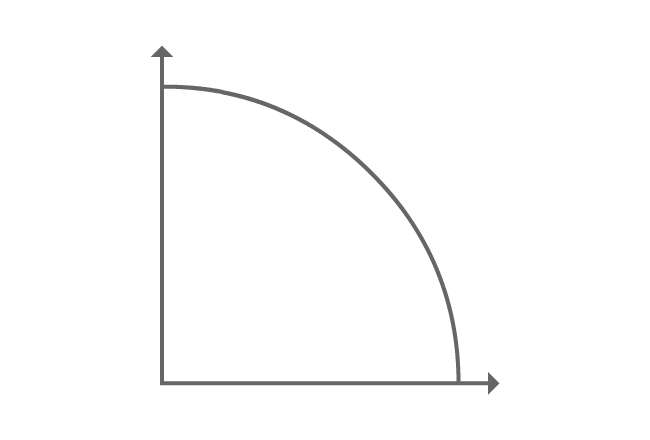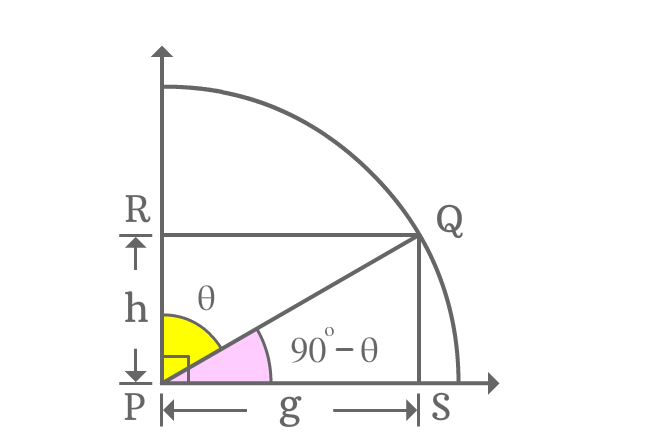# Cofunction identity of cos function

## Formula

Sexagesimal System
$\cos{(90^\circ-\theta)} \,=\, \sin{\theta}$
$\cos{\Big(\dfrac{\pi}{2}-x\Big)} \,=\, \sin{x}$

Theta ($\theta$) is a symbol which represents angle in degrees and $x$ is used to represent angle in radians in this formula.

The angle of cos function is an allied angle of the first quadrant. Hence, the formula is called as first quadrant’s allied angle identity of cos function. The angles of both cos and sin functions are complementary angles. So, this trigonometric identity is popularly called as cofunction identity of cos function.

### ProofConsider first quadrant and a line segment is rotated to an angle and line segment is called as $\overline{PQ}$.

From point $Q$, draw perpendicular lines to horizontal and vertical lines and they meet the rays at points $S$ and $R$ respectively. It constructed two right angled triangles $\Delta QPR$ and $\Delta QPS$ geometrically.

If $\angle QPR = \theta$, then $\angle QPS = 90^\circ-\theta$.

If $PS = g$, then $RQ = g$. Similarly, if $RP = h$, then $QS = h$ due equality and parallelism.

#### Express cos of allied angle$90^\circ-\theta$ is an allied angle of the $\Delta QPS$. Now, write cosine of first quadrant’s allied angle by written it as a ratio of the sides of the triangle.

$\cos{(90^\circ-\theta)} = \dfrac{PS}{PQ}$

The length of the adjacent side ($\overline{PS}$) is $g$ but the length of the hypotenuse is unknown. The length of the opposite side is known. So, the length of the hypotenuse can be evaluated by using Pythagorean theorem.

$\implies \cos{(90^\circ-\theta)} = \dfrac{g}{\sqrt{g^2+h^2}}$

#### Find equivalent value of the fractionCos of allied angle of first quadrant is written as a fraction of the sides in previous step. Now, try to write the fraction of the sides in another equivalent trigonometric function and it can be done by considering $\Delta QPR$.

$\dfrac{g}{\sqrt{g^2+h^2}} = \dfrac{RQ}{PQ}$

According to $\Delta QPR$, the angle of the triangle is theta. The ratio of lengths of $\overline{RQ}$ to $\overline{PQ}$ is sin of angle theta.

$\implies \dfrac{g}{\sqrt{g^2+h^2}} = \sin{\theta}$

#### Cofunction identity of cos function

The above two steps have proved geometrically that

$\cos{(90^\circ-\theta)}$ $=$ $\dfrac{g}{\sqrt{g^2+h^2}}$ $=$ $\sin{\theta}$

$\therefore \,\,\,\,\,\, \cos{(90^\circ-\theta)} \,=\, \sin{\theta}$

It is proved that cos of allied angle of first quadrant is equal to sin of angle. Therefore, the trigonometric identity is known as first quadrant’s allied angle identity of cos function. Actually, the angles of both cos and sin functions are complementary angles. So, the trigonometric identity is generally known as cofunction identity of cos function.

Latest Math Topics
Jun 26, 2023
Jun 23, 2023

Latest Math Problems
Jul 01, 2023
Jun 25, 2023
###### Math Questions

The math problems with solutions to learn how to solve a problem.

Learn solutions

Practice now

###### Math Videos

The math videos tutorials with visual graphics to learn every concept.

Watch now

###### Subscribe us

Get the latest math updates from the Math Doubts by subscribing us.